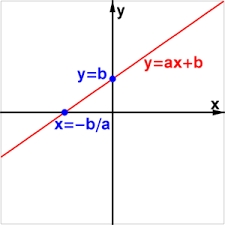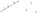# Suppose

Suppose you know that the length of a line segment is 15, x2=6, y2=14 and x1= -3. Find the possible value of y1. Is there more than one possible answer? Why or why not?

Result

y11 =  26
y12 =  2

#### Solution:

$x_{2}=6 \ \\ y_{2}=14 \ \\ x_{1}=-3 \ \\ \ \\ d=15 \ \\ (x_{1}-x_{2})^2 +(y_{1}-y_{2})^2=d^2 \ \\ (-3-6)^2 +(y_{1}-14)^2=15^2 \ \\ (-3-6)^2 +(y_{1}-14)^2=15^2 \ \\ \ \\ \ \\ q^2 -28q +52=0 \ \\ \ \\ a=1; b=-28; c=52 \ \\ D=b^2 - 4ac=28^2 - 4\cdot 1 \cdot 52=576 \ \\ D>0 \ \\ \ \\ q_{1,2}=\dfrac{ -b \pm \sqrt{ D } }{ 2a }=\dfrac{ 28 \pm \sqrt{ 576 } }{ 2 } \ \\ q_{1,2}=\dfrac{ 28 \pm 24 }{ 2 } \ \\ q_{1,2}=14 \pm 12 \ \\ q_{1}=26 \ \\ q_{2}=2 \ \\ \ \\ \text{ Factored form of the equation: } \ \\ (q -26) (q -2)=0 \ \\ \ \\ y_{11}=q_{1}=26$

Checkout calculation with our calculator of quadratic equations.

$y_{12}=q_{2}=2$Our examples were largely sent or created by pupils and students themselves. Therefore, we would be pleased if you could send us any errors you found, spelling mistakes, or rephasing the example. Thank you!

Leave us a comment of this math problem and its solution (i.e. if it is still somewhat unclear...):

Showing 1 comment:Matematik
we make circle k with centre S(x2,y2) and radius r = 15 . Then we make vertical line x= -3 . It make two intersections with circle k thus solutions are two: y11,y12.Tips to related online calculators
For Basic calculations in analytic geometry is helpful line slope calculator. From coordinates of two points in the plane it calculate slope, normal and parametric line equation(s), slope, directional angle, direction vector, the length of segment, intersections the coordinate axes etc.
Looking for help with calculating roots of a quadratic equation?
Do you have a linear equation or system of equations and looking for its solution? Or do you have quadratic equation?
Do you want to convert length units?
Pythagorean theorem is the base for the right triangle calculator.

## Next similar math problems:

1. Three points 2The three points A(3, 8), B(6, 2) and C(10, 2). The point D is such that the line DA is perpendicular to AB and DC is parallel to AB. Calculate the coordinates of D.
2. Set of coordinatesConsider the following ordered pairs that represent a relation. {(–4, –7), (0, 6), (5, –3), (5, 2)} What can be concluded of the domain and range for this relation?
3. Points collinearShow that the point A(-1,3), B(3,2), C(11,0) are col-linear.
4. Parametric formCalculate the distance of point A [2,1] from the line p: X = -1 + 3 t Y = 5-4 t Line p has a parametric form of the line equation. ..
5. CuboidsTwo separate cuboids with different orientation in space. Determine the angle between them, knowing the direction cosine matrix for each separate cuboid. u1=(0.62955056, 0.094432584, 0.77119944) u2=(0.14484653, 0.9208101, 0.36211633)
6. Right angled triangle 2LMN is a right angled triangle with vertices at L(1,3), M(3,5) and N(6,n). Given angle LMN is 90° find n
7. Slope formFind the equation of a line given the point X(8, 1) and slope -2.8. Arrange your answer in the form y = ax + b, where a, b are the constants.
8. Coordinates of a centroindLet’s A = [3, 2, 0], B = [1, -2, 4] and C = [1, 1, 1] be 3 points in space. Calculate the coordinates of the centroid of △ABC (the intersection of the medians).
9. Right triangle from axesA line segment has its ends on the coordinate axes and forms with them a triangle of area equal to 36 square units. The segment passes through the point ( 5,2). What is the slope of the line segment?
10. LineStraight line passing through points A [-3; 22] and B [33; -2]. Determine the total number of points of the line which both coordinates are positive integers.
11. Find the 10Find the value of t if 2tx+5y-6=0 and 5x-4y+8=0 are perpendicular, parallel, what angle does each of the lines make with the x-axis, find the angle between the lines?
12. Find the 5Find the equation with center at (1,20) which touches the line 8x+5y-19=0
13. Two peopleTwo straight lines cross at right angles. Two people start simultaneously at the point of intersection. John walking at the rate of 4 kph in one road, Jenelyn walking at the rate of 8 kph on the other road. How long will it take for them to be 20√5 km apa
14. Vector equationLet’s v = (1, 2, 1), u = (0, -1, 3) and w = (1, 0, 7) . Solve the vector equation c1 v + c2 u + c3 w = 0 for variables c1 c2, c3 and decide weather v, u and w are linear dependent or independent
15. Coordinates of square verticesThe ABCD square has the center S [−3, −2] and the vertex A [1, −3]. Find the coordinates of the other vertices of the square.
16. Angle between vectorsFind the angle between the given vectors to the nearest tenth of a degree. u = (-22, 11) and v = (16, 20)
17. Angle of the body diagonalsUsing vector dot product calculate the angle of the body diagonals of the cube.